# Reducible linear system

(diff) ← Older revision | Latest revision (diff) | Newer revision → (diff)

of ordinary differential equations

A system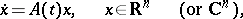(*)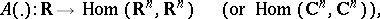that can be transformed into a system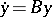with constant coefficients by a change of variables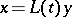, whereis a Lyapunov transformation. If the mapping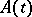is continuous and periodically depends on, then (*) is a reducible system (Lyapunov's theorem). The system (*) is reducible if and only if there is a Lyapunov transformation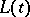and an operatorsuch that every solution of (*) has the form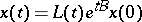(Erugin's criterion).

How to Cite This Entry:
Reducible linear system. Encyclopedia of Mathematics. URL: http://encyclopediaofmath.org/index.php?title=Reducible_linear_system&oldid=18924
This article was adapted from an original article by V.M. Millionshchikov (originator), which appeared in Encyclopedia of Mathematics - ISBN 1402006098. See original article